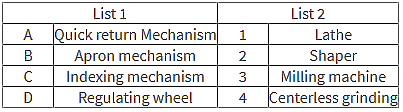Dynamic Analysis of Four Bar Mechanism

Which of the following is an inversion of four bar kinematic chain?
Inversions of Four Bar Chain: Match list 1 with list 2What is the Grashof linkage criterion?
How many equations in total are formed in the process of finding out the forces on each link?
How many forces are acting on each link due to other links?
What is the order of analysis of the four bar linkage?
How many degrees of freedom does a four bar mechanism have?
What is the shortest link for the following category of four bar mechanism?
1. Double crank (s + l < p + q)
2. Double rocker (s + l < p + q)